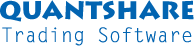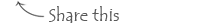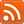#twitter-follow { border: 0;position: fixed; top: 240px; left:0;} #live-support { border: 0;position: fixed; top: 370px; left:0;} #knowledge-base { border: 0;position: fixed; top: 170px; left:0;}

## Anchored Indicator

by The trader, 5032 days agoThis function calculates the number of bars, value, sum, average and rate of return of a specified vector and using reference points. For example, if you use the rate of return indicator (ROC), the function accepts as input a vector and a lookback period (number of bars). If we choose 10 as lookback period and we are in the bar number 20, we then will calculate the rate of return using the current bar (20) and the bar number (10). On the next bar (21), the reference bar will move also to the bar number (11).
This function instead uses a fixed reference bar. This fixed reference bar is calculated using a rule you provide as an input to the function.

Let us say, you want to use a fixed bar that is calculated using the following rule: rsi(14) > 70. The bars where the RSI rule is met will be chosen as reference bars. Therefore, the lookback period used to calculate the ROC will change and will not be fixed as it is using the standard functions. Each time the rule is met the lookback period will be re-initialized (set to zero).

The function has three inputs: The rule used to calculate the reference point, the vector to use for the calculation, and a string that defines the type of calculation that will be made.
The third input can accept the following values:
nb: Calculates the number bars
value: Calculates the value of the provided vector on the bar the last time the rule was true
sum: Calculates the sum
avg: Calculates the average
roc: Calculates the rate of return

-----
Example: anchor(rsi(14) > 70, 1, 'nb');

This function will calculate the number of bars since the RSI rule was true.
In this particular case, the function could be translated to: barssince(rsi(14) > 70);

What is this?

 Type: Trading Indicator Object ID: 301 Country: All Market: All Style: Technical Analysis

 Related objectsEmpty
Number of reviews
Average rate
Click to rate this item
Number of rates the current object received
Report an object
if you can't run it for example or if it contains errors
Click to report this object

 Random Blog Posts How scripts communicate with each other How to simulate options strategies Organizing Trading Objects Creating a download item: Initial Jobless Claims How to search for a download item Trading orders - Part 2 Trading Orders - Part 1 The average maximum drawdown metric Show All

Number of reviews
Average rate
Click to rate this item
Number of rates the current object received
Report an object
if you can't run it for example or if it contains errors
Click to report this objectSupport Contact Us Trading Forum How-to Lessons Manual Company About Us Privacy Terms of Use Copyright © 2023 QuantShare.comTrading financial instruments, including foreign exchange on margin, carries a high level of risk and is not suitable for all investors. The high degree of leverage can work against you as well as for you. Before deciding to invest in financial instruments or foreign exchange you should carefully consider your investment objectives, level of experience, and risk appetite. The possibility exists that you could sustain a loss of some or all of your initial investment and therefore you should not invest money that you cannot afford to lose. You should be aware of all the risks associated with trading and seek advice from an independent financial advisor if you have any doubts.